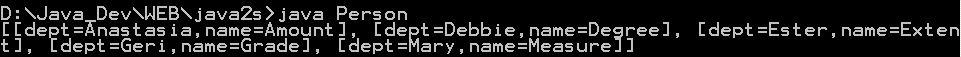This is a very fast, non-cryptographic hash suitable for general hash-based lookup. : Hash Code « Development Class « Java

This is a very fast, non-cryptographic hash suitable for general hash-based lookup.

```
/*
* Copyright 2008-2010 the T2 Project ant the Others.
*
* you may not use this file except in compliance with the License.
* You may obtain a copy of the License at
*
*
* Unless required by applicable law or agreed to in writing, software
* WITHOUT WARRANTIES OR CONDITIONS OF ANY KIND, either express or implied.
* See the License for the specific language governing permissions and
*/
//package org.t2framework.commons.util;

/**
* This is a very fast, non-cryptographic hash suitable for general hash-based
* lookup. See http://murmurhash.googlepages.com/ for more details.
*
* <p>
* The C version of MurmurHash 2.0 found at that site was ported to Java by
* Andrzej Bialecki (ab at getopt org).
* </p>
*
* This is suitable for 32bit application, if 64bit we need to improve it.
*
*/
public class MurmurHashFunction extends AbstractHashFunction {

public int hash(byte[] data, int length, int seed) {
int m = 0x5bd1e995;
int r = 24;
int h = seed ^ length;
int len_4 = length >> 2;

for (int i = 0; i < len_4; i++) {
int i_4 = i << 2;
int k = data[i_4 + 3];
k = k << 8;
k = k | (data[i_4 + 2] & 0xff);
k = k << 8;
k = k | (data[i_4 + 1] & 0xff);
k = k << 8;
k = k | (data[i_4 + 0] & 0xff);
k *= m;
k ^= k >>> r;
k *= m;
h *= m;
h ^= k;
}

// avoid calculating modulo
int len_m = len_4 << 2;
int left = length - len_m;

if (left != 0) {
if (left >= 3) {
h ^= (int) data[length - 3] << 16;
}
if (left >= 2) {
h ^= (int) data[length - 2] << 8;
}
if (left >= 1) {
h ^= (int) data[length - 1];
}

h *= m;
}

h ^= h >>> 13;
h *= m;
h ^= h >>> 15;

return h;
}
}
abstract class AbstractHashFunction implements HashFunction {

public int hash(byte[] bytes, int initval) {
return hash(bytes, bytes.length, initval);
}

public int hash(byte[] bytes) {
return hash(bytes, bytes.length, -1);
}

}
interface HashFunction {

int hash(byte[] bytes);

int hash(byte[] bytes, int initval);

int hash(byte[] bytes, int length, int initval);
}

```

Related examples in the same category

 1 Computing hash codes 2 A hash-code generator and a collection of static hash-code generation methods. 3 MD5 hash generator 4 Hash 32 String 5 Hash 64 String 6 MD5 hashing: Encodes a string 7 MD5 String 8 Hash Code Builder9 HashCode generation10 Get hash code for primitive data types 11 Return as hash code for the given object 12 Null Safe Hash Code 13 A very efficient java hash algorithm, based on the BuzHash algoritm 14 Easy implementation of hashCode 15 An implementation of the HMACT64 keyed hashing algorithm 16 Gets the hash code of an object returning zero when the object is null 17 Unify Hash 18 Secure Hash 19 FNV Hash 20 Jenkins Hash 21 Concurrent Cuckoo hashing using lock striping. Uses R/W locks for resizing. Exercise solution. 22 Concurrent Cuckoo hashing using lock striping. 23 encode Hex 24 Fowler/Noll/Vo hash algorhythm 25 Produces 32-bit hash for hash table lookup. (Jenkins Hash Function) 26 Key Value Hash 27 Paul Hsieh's Hash Function. 28 An extension of WeakReference that implements a sane equals and hashcode method. 29 Dual Key Hash Map 30 A hash map with int key and int values. 31 null Safe Equals and Hash 32 Generates a hash code for a given source code. 33 AtkinsonHash utility class implements the hash algorithm used by HyperCard's ask password command. 34 Hash Code Assist35 An advanced hash table supporting configurable garbage collection semantics of keys and values 36 Hash string